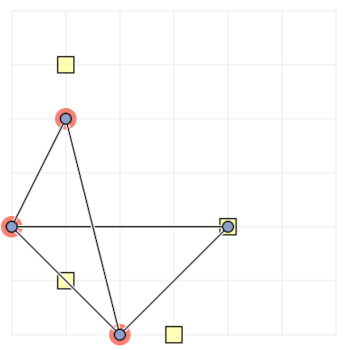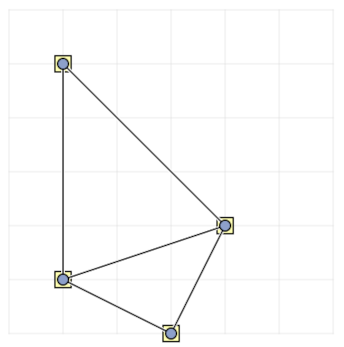## Crossing-Minimal Point-Set Embedding

We shall hold the Graph Drawing Challenge in a format similar to a typical programming contest. At the start of the challenge, teams of contestants will receive the collection of challenge graphs. After one hour, the teams will submit their final drawings and the team with the highest cumulative score wins.

Teams will be allowed to use any combination of software and human interaction systems to produce the best drawings. To accommodate both teams wishing to prepare for the challenge and teams wishing simply to participate, with no preparation, we will be providing, in advance, a small set of graph visualization tools. These tools are not necessarily meant to solve the problems at hand but are there to help the teams manually draw and manipulate the graphs. To further the development of new tools and to help promote tools already in existence, teams are also welcome and highly encouraged to create and bring their own software packages.

There will be two categories in the challenge that are judged independently:

• Automatic: This category is for teams using their own tool. Since we assume that the tool contains special algorithms to solve the challenge automatically, these teams will receive larger challenge graphs. Manual fine-tuning is allowed.

• Manual: This category is for teams using the provided graph editor. The graph editor does not contain any specific algorithm to solve the challenge. It allows only to change vertex locations. This category is for creating manual solutions without help of an automatic algorithm. Teams in this category will receive smaller challenge graphs. The tool is available in the submission system.

The challenge focuses on minimizing crossings on a given set of points. The input therefore consists of a graph and a set of points (at least as many as vertices of the graph). The goal is to assign the vertices of the graph to the given points, such that the number of crossings in the resulting drawing is as low as possible (see below on how degeneracies are handled).

Here is an overview of the rules for the challenge:

• The challenge will take place for one hour during the Graph Drawing Symposium.
• Teams may consist of up to three participants each. Each team should bring their own computers and/or software tools to the challenge.
• Software tools for manually solving the challenge will be provided for each team with time available prior to the challenge to set-up and practice with the system.
• At the start of the challenge, contestants will receive a collection of graphs in the format described below. Note that the point set will use integer coordinates, but is not necessarily in general position.
• For each graph, the team submitting the drawing with the smallest number of crossings receives the highest score. Scores for other submissions of the same graph shall be weighed with respect to this value.
• The team with the highest total score over all graphs wins.

## Counting crossings

The basic idea is to count the number of crossing edge pairs. Yet, we must be careful with collinearities. Specifically, a vertex lying on an edge (that it is not incident to) gives vertex-edge resolution of zero and as such is penalized strongly. Our definition ensures that any (injective) mapping of vertices to points achieves a finite score and can thus be considered “valid”.

A drawing of a graph with $$n$$ vertices and $$m$$ edges $$e_1,\ldots,e_m$$ has the following number of crossings (assuming the vertices are mapped injectively onto the given points):

$$\sum_{i=1}^m \sum_{j=i+1}^m \mathrm{cross}(e_i,e_j)$$

where $$\mathrm{cross}(e,e')$$ is a function interprets both edges as closed line segments and returns the following:

• $$0$$ if there is no common point, or there is exactly one common point and that point is an endpoint of both segments;
• $$1$$ if there is exactly one a common point and that point is an interior point of both segments;
• $$n$$ otherwise (an endpoint of one segment is an interior point of the other segment; this is implied by segments overlapping, thereby having multiple points in common).

Any drawing that maps distinct vertices to the same point (or to coordinates not in the given set) are considered “invalid” and are defined to have an infinite number of crossings.

To illustrate the behavior of the $$\mathrm{cross}$$ function, we give the following seven examples. Note that the first three examples are fairly standard in counting crossings.Case 1a: no common point, so these two edges have a $$\mathrm{cross}$$ value of $$0$$.Case 1b: the only common point is the shared endpoint of both edges (white circle), so these two edges have a $$\mathrm{cross}$$ value of $$0$$.Case 2: the only common point is interior to both edges, so these two edges have a $$\mathrm{cross}$$ value of $$1$$.Case 3a: the lower white square lies on top of the edge connecting the black dots, so these two edges have a $$\mathrm{cross}$$ value of $$n$$.Case 3b: the lower white square lies on top of the edge connecting the black dots (and the upper black dot lies on top of the edge connecting the white squares), so these two edges have a $$\mathrm{cross}$$ value of $$n$$.Case 3c: both black dots lie on top of the edge connecting the white squares, so these two edges have a $$\mathrm{cross}$$ value of $$n$$.Case 3d: though the two edges share a vertex (white circle) the black dot lies on top of the edge connecting the shared vertex to the white square, so these two edges have a $$\mathrm{cross}$$ value of $$n$$.

## Submission tool

Go to the provided tool in the submission system

## File Format

For the GD Contest 2023, a JSON format described below will be used. The contest graphs will be provided in this format and the final submissions should be prepared using the same format. A valid JSON file consists of the following entries:

• nodes: An array with one element per node of the graph. Each element has the following entries:
• id: The id of the node. Has to be an integer between 0 and #nodes-1.
• x: The x-coordinate of the node. Has to be an integer between 0 and width.
• y: The y-coordinate of the node. Has to be an integer between 0 and height.
• edges: An array with one element per edge of the graph. Each element has the following entries:
• source: The id of the source node.
• target: The id of the target node.
• points: An array with one element per point of the given point set. Each element has the following entries:
• id: The id of the point. Has to be an integer between 0 and #points-1.
• x: The x-coordinate of the point. Has to be an integer between 0 and width.
• y: The y-coordinate of the point. Has to be an integer between 0 and height.
• width (optional): The maximum x-coordinate of the grid. Has to be a non-negative integer. If unspecified, the width is set to 1,000,000.
• height (optional): The maximum y-coordinate of the grid. Has to be a non-negative integer. If unspecified, the height is set to 1,000,000.

## Sample File

Below is a simple example:


{ "nodes": [
{ "id": 0, "x": 1, "y": 0 },
{ "id": 1, "x": 0, "y": 2 },
{ "id": 2, "x": 2, "y": 2 },
{ "id": 3, "x": 1, "y": 4 }],
"edges": [
{ "source": 0, "target": 1 },
{ "source": 0, "target": 2 },
{ "source": 1, "target": 2 },
{ "source": 1, "target": 3 },
{ "source": 2, "target": 3 }],
"points": [
{ "id": 0, "x": 1, "y": 1},
{ "id": 1, "x": 3, "y": 0},
{ "id": 2, "x": 4, "y": 2},
{ "id": 3, "x": 1, "y": 5}],
"width": 6,
"height": 6
}



The left drawing below corresponds to this input file. This drawing is has 1 crossing, but is invalid because three nodes do not lie on the point set. The right drawing shows an optimal solution where all nodes lie on the point set and there are 0 edge crossings.## How to participate

The Live Challenge will be part of the 31st International Symposium on Graph Drawing and Network Visualization, and will take place on

Wednesday, September 20, 18:00-19:00 CEST/UTC+2.

If you wish to participate in the manual category, you will attempt to solve the problems using the supplied tool.

If you wish to participate in the automatic category, you can use any software you wish to solve the problems, including the supplied tool. However, problems instances will be much larger than in the manual category.

You may use at most one computer per team.

Remote participation is possible, in case the conference itself is organized as a hybrid (or online) conference.

The challenge will be run through an online submission system. You can already visit the system and try out the sample graph to get yourself familiar with the provided tool.